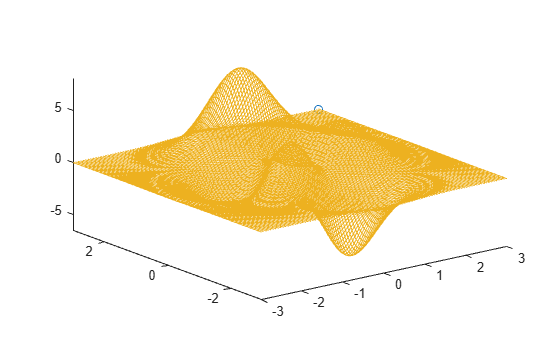comet3

3-D comet plot

Description

comet3(z) displays a comet plot of the data specified in z versus x- and y-values matching the indices of z. A comet is an animation of a marker (head) and a line (tail) tracing a growing line over the data points. The tail is a solid line that traces the entire function.

example

comet3(x,y,z) displays a comet plot of z versus x and y.

example

comet3(x,y,z,p) specifies the comet body length. The comet body is a trailing segment in a different color that follows the head before fading. The body length is p*length(y), where p is a scalar in [0, 1).

example

comet3(ax,x,y,z,p) plots the comet into the axes specified by ax.

Examples

collapse all

Create a comet plot of the data in z versus the data in x and y. Use the peaks function to load x, y, and z data in matrix forms. Convert the data into vector arrays. Then, plot the data.

[xmat,ymat,zmat] = peaks(100);
xvec = xmat(:);
yvec = ymat(:);
zvec = zmat(:);
comet3(xvec,yvec,zvec)Create a comet plot and specify the comet body length by setting the scale factor input p. The comet body is a trailing segment in a different color that follows the head before fading.

Use the peaks function to load x, y, and z data in matrix forms. Convert the data into vector arrays. Specify p as 0.5 so that the body length is 0.5*length(y). Then, plot the data.

[xmat,ymat,zmat] = peaks(100);
xvec = xmat(:);
yvec = ymat(:);
zvec = zmat(:);
p = 0.5;
comet3(xvec,yvec,zvec,p)Create two comet plots in a tiled chart layout by specifying the target axes for each plot.

Use the peaks function to load x, y, and z data in matrix forms. Convert the data into vector arrays. Specify the body length scale factor p as 0.25 so that the body length is 0.5*length(y).

[xmat,ymat,zmat] = peaks(50);
xvec = xmat(:);
yvec = ymat(:);
zvec = zmat(:);
p = 0.25;

Store the two Axes objects as ax1 and ax2. Specify the target axes for each comet plot by including the Axes object as the first input argument to comet.

tiledlayout(1,2);
ax1 = nexttile;
ax2 = nexttile;

comet3(ax1,xvec,yvec,zvec,p)
comet3(ax2,yvec,xvec,zvec,p)Input Arguments

collapse all

z-values, specified as a vector. To plot against specific x- and y-values you must also specify x and y.

x-values, specified as a vector. x, y, and z must be the same size. If you specify geographic axes for ax, then x represents latitude in degrees.

y-values, sp0ecified as a vector. x, y, and z must be the same size. If you specify geographic axes for ax, then y represents longitude in degrees.

Comet body length scale factor, specified as a value in the interval [0, 1). The comet body length is p*length(y).

Target axes, specified as an Axes object or GeographicAxes object.

Limitations

• Comet plots do not support data tips.